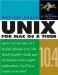# Creating and Using Functions

Creating and Using Functions

Functions are series of commands that have a name much like miniature scripts that can be stored inside another script. Functions make your code easier to understand and maintain. If you have a series of commands that you use more than once in your script, or that you want to use in more than one script, consider putting it in a function. You give the set of commands a name, and then in your script you use the name instead of repeating all of the command lines that the name refers to. You can pass arguments to functions in a manner similar to passing arguments to commands.

While reading the following task, refer to Figure 9.36 , which is a code listing of a script that uses a function, and Figure 9.37 , which shows output from the script.

##### Figure 9.36. Code listing of a script that uses a function.
` #!/bin/sh # The script uses a function magic=77 guess=0 # define a function called "ask" ask () {     echo -n "Pick a number between 1 and 100: "     read guess } while [ \$guess -ne \$magic ] ; do     ask     if [ \$guess -lt \$magic ] ; then           echo "Try a higher number."     elif [ \$guess -gt \$magic ] ; then           echo "Try a lower number"     else           echo "Hey! You got it!"     fi done `

##### Figure 9.37. Output from the script in Figure 9.36.
` localhost:~ vanilla\$  ./function.sh  Pick a number between 1 and 100:  13  Try a higher number. Pick a number between 1 and 100:  50  Try a higher number. Pick a number between 1 and 100:  75  Try a higher number. Pick a number between 1 and 100:  88  Try a lower number Pick a number between 1 and 100:  80  Try a lower number Pick a number between 1 and 100:  79  Try a lower number Pick a number between 1 and 100:  78  Try a lower number Pick a number between 1 and 100:  77  Hey! You got it! localhost:~ vanilla\$ `

To create a function:

 1 name () { The function name can be any combination of letters , numbers , dashes, and underscore characters as long as it isn't the same as a predefined or built-in shell command, such as if , while , and so forth (see man builtin ). 2 Enter a series of command lines. The body of the function is a series of command lines. Indent the commands in the function to make the script easier to read. 3 } The } ends the function. Now you can use the function at any point farther on in your script, as if you have added a new command to the Bourne shell language. (You cannot use a function in a script at a point earlier than where the function is defined.)

Functions can take arguments just as a script or command does.

To use arguments in a function:

• Use the special variables for arguments in the function.

The special variables you have used for script arguments all work inside a function. When used inside a function, they refer to the arguments used with the function, not the script around the function. So the \$3 inside a function is the third argument to the function, not the third argument to the script.

Figure 9.38 is a code listing of a script using a function that takes arguments, and Figure 9.39 is output from that script.

##### Figure 9.38. Code listing of a script using a function that takes arguments.
` #!/bin/sh # The script uses a function that takes arguments magic=63 a=0 b=0 sum=0 ask () {        echo -n "Enter two integers: "        read a b } add () {        sum=`expr  + ` } while [ \$magic -ne \$sum ] ; do        ask        add \$a \$b        if [ \$sum -lt \$magic ] ; then             echo "Try HIGHER."        elif [ \$sum -gt \$magic ] ; then             echo "Try LOWER."        else             echo "VERY GOOD. \$a + \$b = \$magic which is the magic number."   fi done `

##### Figure 9.39. Output of the script in Figure 9.38.
` localhost:~ vanilla\$  ./function.sh  Enter two integers:  23 37  Try HIGHER. Enter two integers:  30 40  Try LOWER. Enter two integers:  29 40  Try LOWER. Enter two integers:  27 37  Try LOWER. Enter two integers:  25 37  Try HIGHER. Enter two integers:  26 37  VERY GOOD. 26 + 37 = 63 which is the magic number. localhost:~ vanilla\$ `Tip

• If you have a function or functions that you want to use in more than one script, you should put the function(s) in a separate file and read that file into your script(s) using the . command:

. file

file must be a path to the file you want to read. If it is simply a filename, then the file must be in your current directory when you execute the script. The script will read the named file and execute its contents as if they were typed into the script at this point.

Of course the Bourne shell man page, man sh , will at least give you a good overview of what else there is to learn, although it may not be the best guide for a beginner to actually work from.

Here are two online tutorials:

• Unix Bourne Shell Scripting (http://unix.about.com/library/course/blshscript-outline.htm)

• Steve Parker's Web site (http://steve- parker .org/sh/sh.shtml)

If you use the bash shell, you should read Learning the bash Shell , Second Edition, by Cameron Newham and Bill Rosenblatt (O'Reilly; www.oreilly.com/catalog/bash2).Unix for Mac OS X 10.4 Tiger: Visual QuickPro Guide (2nd Edition)
ISBN: 0321246683
EAN: 2147483647
Year: 2004
Pages: 161
Authors: Matisse Enzer

Similar book on Amazon